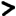Plasma GitLab Archive Projects Blog KnowledgePlasma Project:
 HomeManualFAQSlidesPerfReleases# Pfs_dn_internal

```/* \$Id: pfs_dn_internal.x 450 2011-10-11 12:30:40Z gerd \$ -*- c -*- */

```
Internal stuff.

```
#ifndef PFS_DN_INTERNAL_X
#define PFS_DN_INTERNAL_X

#include "pfs_types.x"

```

# `Datanode_ctrl`

The `Datanode_ctrl` program is running on each datanode, but only invoked by the coordinator to push and revoke safetrans tickets.

```

program Datanode_ctrl {
version V1 {

```

## `null`

```
void null(void) = 0;

```

## `reset_all_safetrans`

```
void reset_all_safetrans(void) = 1;
```
Revokes all safetrans tickets. This is called when the coordinator starts up.

## `cancel_safetrans`

```
void cancel_safetrans(hypers) = 2;
```
Cancel the safetrans ticket with these `st_id`

## `safetrans`

```
void safetrans(enable_tickets) = 3;
```
`safetrans(st_id, st_tmo, st_secret)`: Enables all safetrans tickets with ID `st_id`. The secret `st_secret` is used for securing the ticket system.

```
} = 1;
} = 0x8000d002;

```

# `Datanode_io`

The `Datanode_io` program is running in the I/O processes of the datanodes

```
program Datanode_io {
version V1 {
```

## `null`

```
void null(void) = 0;

```

## `read`

```
void read(int, hyper, int, int) = 1;
```
`read(slot, block, pos, len)`: Reads the block (or part of it) to the shm `slot`

## `read_shm`

```
void read_shm(dn_channel_shm_obj, hyper, int, int) = 7;
```
`read_shm(shm, block, pos, len)`: Reads the block (or part of it) to the passed shm object. It is an error if the oject is not large enough.

## `write`

```
void write(int, hyper) = 2;
```
`write(slot, block)`: Writes the contents of `slot` to `block`

## `write_shm`

```
void write_shm(dn_channel_shm_obj, hyper) = 8;
```
`write_shm(shm, block)`: Writes the contents of `shm` to `block`. Exactly blocksize bytes are written.

## `copy`

```
void copy(hyper, mediumstring, mediumstring, hyper, ticket, int) = 3;
```
```copy(block, dest_node, dest_identity, dest_block, dest_ticket, slot)```

## `sync`

```
void sync(void) = 4;
```
syncs everything to disk

## `size`

```
hyper size(void) = 5;
```
same as in `Datanode`

## `identity`

```
mediumstring identity(void) = 6;
```
same as in `Datanode`

```
} = 1;
} = 0x8000d003;

```

# `Datanode_discover`

This is a UDP program used for discovering datanodes (multicasting), and for monitoring the liveliness of datanodes.

```
program Datanode_discover {
version V1 {
```

## `null`

```
void null(void) = 0;

```

## `identity`

```
mediumstring identity(mediumstring) = 1;
```
Returns the identity of this node (an ID which is assigned anew when the datanode is initialized). The arg is the clustername. If the node belongs to the wrong cluster, this RPC must be silent.

```    } = 1;
} = 0x8000d004;

#endif
```